# PHP: Comparison Operators

## Description

In PHP, comparison operators take simple values (numbers or strings) as arguments and evaluate to either TRUE or FALSE.

Here is a list of comparison operators.

Operator Name Example Result
= = Equal \$x == \$y TRUE if \$x is exactly equal to \$y
= = = Identical \$x === \$y TRUE if \$x is exactly equal to \$y, and they are of the same type.
!= Not equal \$x != \$y TRUE if \$x is exactly not equal to \$y.
<> Not equal \$x <> \$y TRUE if \$x is exactly not equal to \$y.
!== Not identical \$x !== \$y TRUE if \$x is not equal to \$y, or they are not of the same type.
< Less than \$x < \$y TRUE if \$x (left-hand argument) is strictly less than \$y (right-hand argument).
> Greater than \$x > \$y TRUE if \$x (left hand argument)  is strictly greater than \$y (right hand argument).
<= Less than or equal to \$x <= \$y TRUE if \$x (left hand argument) is less than or equal to \$y (right hand argument).
>= Greater than or equal to \$x >= \$y TRUE if \$x is greater than or equal to \$y.

Pictorial presentation of Equal (==) operator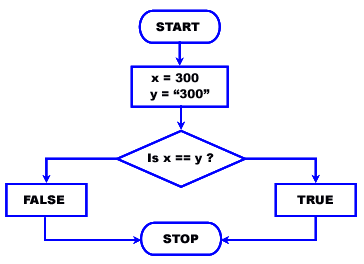Test Equal (==) operator

The following php codes return true though the type of \$x and \$y are not equal (the first one is integer type and the second one is character type) but their values are equal.

``````<?php
\$x = 300;
\$y = "300";
var_dump(\$x == \$y);
?>``````

Output :

` bool(true)`

Pictorial presentation of Strict equal (===) operator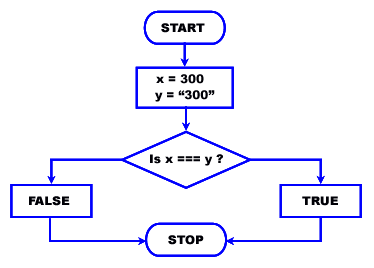Test Strict equal (===) operator

The following php codes returns false as the strict equal operator will compare both value and type of \$x and \$y.

``````<?php
\$x = 300;
\$y = "300";
var_dump(\$x === \$y);
?>``````

Output :

bool(false)

Pictorial presentation of Not equal(!=)/(<>) operator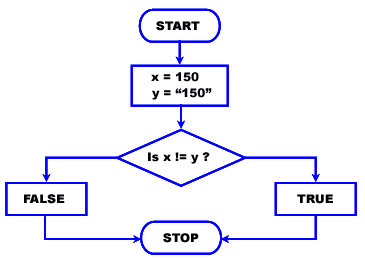Test Not equal (!=) / (<>) operator

The following php codes return false though the type of \$x and \$y are not equal (the first one is integer type and the second one is character type) but their values are equal.

``````<?php
\$x = 150;
\$y = "150";
var_dump(\$x != \$y);
?>``````

Output of the example

`bool(false) `

Test Not identical (!==) operator

The following php codes return true though their values are equal but the type of \$x and \$y are not equal (the first one is integer type and the second one is character type).

``````<?php
\$x = 150;
\$y = "150";
var_dump(\$x !== \$y);
?>``````

Output :

`bool(true) `

Pictorial presentation of Greater than(>) operator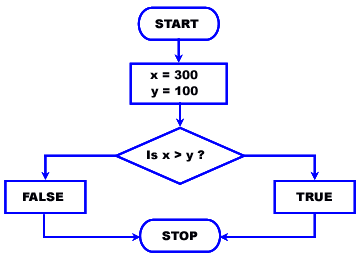Test Greater than(>) operator

The following php code return true as the value of \$x is greater than \$y.

``````<?php
\$x = 300;
\$y = 100;
var_dump(\$x>\$y);
?>``````

Output :

`bool(true) `

Pictorial presentation of Greater than or equal (>=)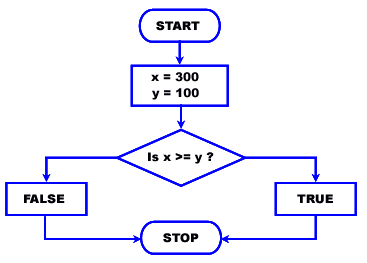Test Greater than or equal (>=) operator

The following php codes return true as the value of \$x is equal to \$y.

``````<?php
\$x = 300;
\$y = 100;
var_dump(\$x>=\$y);
?>``````

Output :

`bool(true) `

Pictorial presentation of Less than (<) operator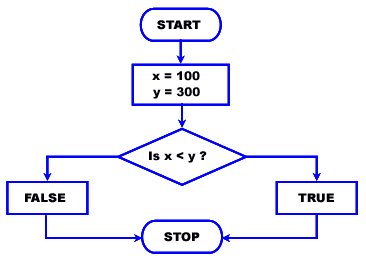Test Less than (<) operator

The following php codes return true as the value of \$x is less than \$y.

``````<?php
\$x = 100;
\$y = 300;
var_dump(\$x<\$y);
?>``````

Output :

`bool(true) `

Pictorial presentation of Less than or equal (<=) operator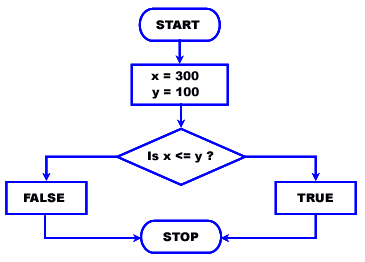Test Less than or equal (<=) operator

The following PHP codes return false as the value of \$x is greater than \$y.

``````<?php
\$x = 300;
\$y = 100;
var_dump(\$x<=\$y);
?>``````

Output :

`bool(false) `

PHP variables

Previous: Arithmetic Operators
Next: Logical Operators

﻿

## PHP: Tips of the Day

Variables can be incremented or decremented by 1 with ++ or --, respectively. They can either precede or succeed variables and slightly vary semantically, as shown below.

Example:

```\$i = 1;
echo \$i; // Prints 1
// Pre-increment operator increments \$i by one, then returns \$i
echo ++\$i; // Prints 2
// Pre-decrement operator decrements \$i by one, then returns \$i
echo --\$i; // Prints 1
// Post-increment operator returns \$i, then increments \$i by one
echo \$i++; // Prints 1 (but \$i value is now 2)
// Post-decrement operator returns \$i, then decrements \$i by one
echo \$i--; // Prints 2 (but \$i value is now 1)
```